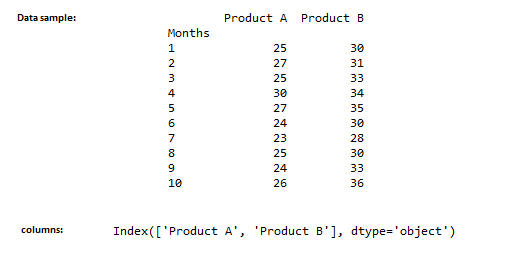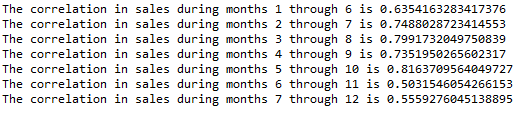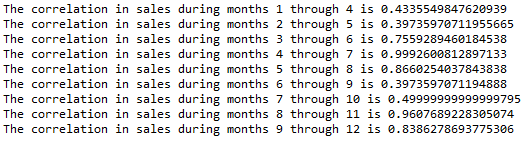Open in App
Not now

# How to Calculate Rolling Correlation in Python?

• Last Updated : 29 Jan, 2022

Correlation generally determines the relationship between two variables. The rolling correlation measure the correlation between two-time series data on a rolling window Rolling correlation can be applied to a specific window width to determine short-term correlations.

## Calculating Rolling Correlation in Python

Let’s use sales data of two products A and B in the last 60 months to calculate the rolling correlation. Pandas package provides a function called rolling.corr() to calculate the rolling correlation.

Syntax:

`data1.rolling(width).corr(data2)`

Where,

• data1, data2 – data/column of interest (type series)
• width – Rolling window width (int)

Note: The width of the rolling window should be 3 or greater in order to calculate correlations.

Data Used:

## Python3

 `# import pandas module``import` `pandas as pd` `# read the data``data ``=` `pd.read_csv(``'product_sales.csv'``)` `# display top 10 rows``print``(data.head(``10``))` `# display column names``print``(data.columns)`

Output:Example 2:

Here, we used the window width of 6, which shows the successive 6 months rolling correlation. We could see a significant correlation between two products sales any sudden dip or rise in correlation signals an unusual event, that caused the dip.

## Python3

 `data[``'Product A'``].rolling(``6``).corr(data[``'Product B'``])` `# formatting the output``k ``=` `1``for` `i, j ``in` `enumerate``(data[``'Product A'``].rolling(``6``).corr(data[``'Product B'``])):``    ``if` `(i >``=` `5` `and` `i < ``12``):``        ``print``(f'The correlation ``in` `sales during months\``        ``{k} through {i``+``1``} ``is` `{j}')``        ``i ``=` `0``        ``k ``+``=` `1`

Output:Now’s let us try the same for 3-month correlation as shown below,

Example 3:

## Python3

 `data[``'Product A'``].rolling(``3``).corr(data[``'Product B'``])` `# formatting the output``k ``=` `1``for` `i, j ``in` `enumerate``(data[``'Product A'``].rolling(``3``).corr(data[``'Product B'``])):``    ``if` `(i >``=` `3` `and` `i < ``12``):``        ``print``(``            ``f'The correlation ``in` `sales during months {k} \``            ``through {i``+``1``} ``is` `{j}')``        ``i ``=` `0``        ``k ``+``=` `1`

Output:My Personal Notes arrow_drop_up